Download Presentation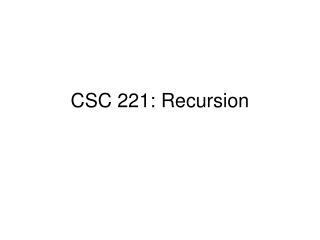CSC 221: Recursion

# CSC 221: Recursion - PowerPoint PPT Presentation

CSC 221: Recursion. Recursion: Definition. Function that solves a problem by relying on itself to compute the correct solution for a smaller version of the problem Requires terminating condition : Case for which recursion is no longer needed. Recursion: Induction Basis.I am the owner, or an agent authorized to act on behalf of the owner, of the copyrighted work described.
Download Presentation## CSC 221: Recursion

An Image/Link below is provided (as is) to download presentation

Download Policy: Content on the Website is provided to you AS IS for your information and personal use and may not be sold / licensed / shared on other websites without getting consent from its author.While downloading, if for some reason you are not able to download a presentation, the publisher may have deleted the file from their server.

- - - - - - - - - - - - - - - - - - - - - - - - - - E N D - - - - - - - - - - - - - - - - - - - - - - - - - -
Presentation Transcript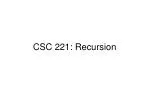### CSC 221: Recursion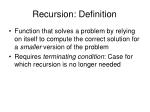Recursion: Definition
• Function that solves a problem by relying on itself to compute the correct solution for a smaller version of the problem
• Requires terminating condition: Case for which recursion is no longer needed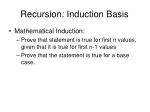Recursion: Induction Basis
• Mathematical Induction:
• Prove that statement is true for first n values, given that it is true for first n-1 values
• Prove that the statement is true for a base case.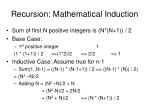Recursion: Mathematical Induction
• Sum of first N positive integers is (N*(N+1)) / 2
• Base Case:
• 1st positive integer 1

(1 * (1+1)) / 2 =>(1*2)/2 => 2/2 => 1

• Inductive Case: Assume true for n-1
• Sum(1..N-1) = ((N-1) * (N-1+1)) / 2 => ((N-1) * (N)) / 2)

= (N2 –N)/2

• Adding N = (N2 –N)/2 + N

= (N2-N)/2 + 2N/2

= (N2 + N)/2 => (N * (N+1)) / 2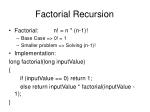Factorial Recursion
• Factorial: n! = n * (n-1)!
• Base Case => 0! = 1
• Smaller problem => Solving (n-1)!
• Implementation:

long factorial(long inputValue)

{

if (inputValue == 0) return 1;

else return inputValue * factorial(inputValue - 1);

}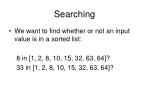Searching
• We want to find whether or not an input value is in a sorted list:

8 in [1, 2, 8, 10, 15, 32, 63, 64]?

33 in [1, 2, 8, 10, 15, 32, 63, 64]?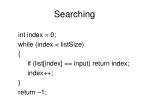Searching

int index = 0;

while (index < listSize)

{

if (list[index] == input) return index;

index++;

}

return –1;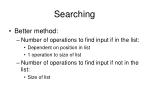Searching
• Better method:
• Number of operations to find input if in the list:
• Dependent on position in list
• 1 operation to size of list
• Number of operations to find input if not in the list:
• Size of list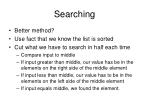Searching
• Better method?
• Use fact that we know the list is sorted
• Cut what we have to search in half each time
• Compare input to middle
• If input greater than middle, our value has be in the elements on the right side of the middle element
• If input less than middle, our value has to be in the elements on the left side of the middle element
• If input equals middle, we found the element.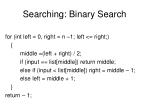Searching: Binary Search

for (int left = 0, right = n –1; left <= right;)

{

middle =(left + right) / 2;

if (input == list[middle]) return middle;

else if (input < list[middle]) right = middle – 1;

else left = middle + 1;

}

return – 1;Searching: Binary Search

8 in [1, 2, 8, 10, 15, 32, 63, 64]?

1st iteration:

Left = 0, Right = 7, Middle = 3, List[Middle] = 10

Check 8 == 10 => No, 8 < 10

2nd iteration:

Left = 0, Right = 2, Middle = 1, List[Middle] = 2

Check 8 == 2 => No, 8 > 2

3rd iteration:

Left = 2, Right = 2, Middle = 2

Check 8 == 8 => Yes, Found It!Searching: Binary Search
• Binary Search Method:
• Number of operations to find input if in the list:
• Dependent on position in list
• 1 operation if middle
• Log2 n operations maximum
• Number of operations to find input if not in the list:
• Log2 n operations maximumRecursive Binary Search
• Two requirements for recursion:
• Same algorithm, smaller problem
• Termination condition
• Binary search?
• Search in half of previous array
• Stop when down to one elementRecursive Binary Search

int BinarySearch(int *list, const int input, const int left, const int right)

{

if (left < right)

{

middle =(left + right) / 2;

if (input == list[middle]) return middle;

else if (input < list[middle]) return BinarySearch(list, input, left, middle-1);

else return BinarySearch(list,input,middle+1,right);

}

return – 1;

}While vs Recursion
• While and Recursion are essentially interchangeable
• Considerations:
• Efficiency
• Simplification of programming
• While they are equivalent, there is not always an obvious while implementation of some functions that are easily implemented with recursionFibonacci Computation
• Fibonacci Sequence:
• 1, 1, 2, 3, 5, 8, 13, 21, 34, 55, 89, …
• Simple definition:
• Fib = 1
• Fib = 1
• Fib[N] = Fib(N-1) + Fib(N-2)Recursive Fibonacci

int fibonacci(int input)

{

if ((input == 0) || (input == 1)) return 1;

else return (fibonacci(input-1) + fibonacci(input-2));

}Iterative Fibonacci

int fibonacci(int input)

{

int first = 1;

int second = 1;

int temp;

for (int k = 0; k < input; k++)

{

temp = first;

first = second;

second = temp + second;

}

return first;

}Efficiency of Recursion
• Recursion can sometimes be slower than iterative code
• Two main reasons:
• Program stack usage
• Result generationTypes of Recursion
• Linear Recursion:
• 1 recursive call per function
• Factorial, Binary Search examples
• Tree Recursion:
• 2 or more recursive calls per function
• Fibonacci ExampleEfficiency of Recursion
• Stack Usage:
• When a function is called by a program, that function is placed on the program call stack:

Returns file data to be used in getData()

getData()

main()

Returns formatted data to be printed in

main()Efficiency of Recursion
• Every stack entry maintains information about the function:
• Where to return to when the function completes
• Storage for local variables
• Pointers or copies of arguments passed inEfficiency of Recursion
• When using recursive functions, every recursive call is added to the stack and it grows fast:
• Fibonacci (5)
• Fibonacci (5) = Fibonacci (4) +

Fibonacci(3)

• Fibonacci (1) in the computation

of Fibonacci(4) is the first time we don’t

have to call the function again.

• Stack entries take up space and

require extra processing

Fibonacci(1)

Fibonacci(2)

Fibonacci(3)

Fibonacci(4)

Fibonacci(5)

main()Efficiency of Recursion
• Another Reason for Slowdowns [Tree Recursion]
• Traditional Recursion doesn’t save answers as it executes
• Fib(5)

= Fib(4) + Fib(3)

= Fib(3) + Fib(2) + Fib(3)

= Fib(2) + Fib(1) + Fib(2) + Fib(3)

= Fib(1) + Fib(0) + Fib(1) + Fib(2) + Fib(3)

= Fib(1) + Fib(0) + Fib(1) + Fib(1) + Fib(0) + Fib(3)

= Fib(1) + Fib(0) + Fib(1) + Fib(1) + Fib(0) + Fib(2) + Fib(1)

= Fib(1) + Fib(0) + Fib(1) + Fib(1) + Fib(0) + Fib(1) + Fib(0) + Fib(1)

• Solution: Dynamic programming – saving answers as you go and reusing themDynamic Programming
• Fibonacci Problem
• We know the upper bound we are solving for
• Ie Fibonacci (60) = 60 different answers
• Generate an array 60 long and initalize to –1
• Everytime we find a solution, fill it in in the array
• Next time we look for a solution, if the value in the array for the factorial we need is not –1, use the value present.Fibonacci Examples
• Three implementations of fibonacci:
• Naive recursion implementation

(worst performance)

• Dynamic Programming recursion implementation (better)
• Iterative implementation

(best)Recursive Datastructures
• Recursion is useful when datastructure is inherently recursive
• Unix directory hierarchy is a tree datastructure

/

/home

/var

/usr

/home/turketwh

/home/turketwh/CS112

/home/turketwh/CS221Directory Traversal
• ls –R in Unix => recursive list
• Potential implementation?
• listDirectory(directory baseDirectory)

{

file[] files = getFiles();

int fileCount = getFileCount();

for (int i = 0; i < fileCount; i++)

{

if (files[I].type == “dir”) listDirectory(file);

else listFile(file);

}

}Recursive Datastructures
• Will see a lot of datastructures that are recursive
• Lists [Atom + Smaller List]
• Trees [ Root + Subtrees]
• Have simple implementations because their functionality can be defined recursively.Towers of Hanoi

Not allowed

General Problem: For any number of boxes, move boxes from start peg to

destination peg. Can never place a bigger box on top of a smaller box.Towers Of Hanoi
• Recursive?
• 1 box from peg 1 to peg 3

1 _ _ _ _ 1

• 2 boxes from peg 1 to peg 3

1 1

2 _ _ 2 1 _ _ 1 2 _ _ 2Towers Of Hanoi
• 3 boxes from peg 1 to peg 3

1

2 2 1 1

3 _ _ 3 _ 1 3 2 1 3 2 _ _ 2 3

1

2 2

1 2 3 1 _ 3 _ _ 3

• For n boxes,
• Solve the n-1 problem from start to the temp peg
• Move the nth box to the destination peg
• Solve the n-1 problem from the temp peg to the destination pegSpace Efficiency
• Factorial(N)
• Factorial(4) =>

= 4*Factorial(3)

= 4 * 3 * Factorial(2)

= 4 * 3 * 2 * Factorial(1)

= 4 * 3 * 2 * 1

• Has to make a maximum of N calls before base case is reached and function returns.
• N activation records will be placed on the stack.
• Since the size of the input is N, this function requires memory that is linearly related to the size of the inputSpace Efficiency
• Fibonacci(N) – Standard recursive implementation
• Fib(5) = Fib(4) + Fib(3)
• For Fib(4), also puts Fib(3),Fib(2), Fib(1) on stack
• For Fib(3), also puts Fib(2), Fib(1) on stack
• Fib(4), Fib(3) compute separately
• Space Efficiency considers maximum amount required at one time – Fib(4) branch in this case
• Requires memory linearly related to input sizeTail Recursion

Tail Recursion: When the results of a recursive call are not used after the recursive call within the calling function.

long factorialHelper(long startValue, long inputValue)

{

if (startValue == 0) return 1;

else if (inputValue == 1) return startValue;

else return factorialHelper(startValue * (inputValue-1), inputValue - 1);

}

long factorial(long inputValue)

{

return factorialHelp(inputValue, inputValue);

}Space Efficiency: Tail Recursion
• Since the results of the recursive call aren’t needed for other computations, the stack frames aren’t needed to hold partial results.
• With each call, new frame replaces old frame.
• The compiler handles these optimizations.
• Requires constant amount of memory and is not dependent on the size of the input.Space Efficiency: Tail Recursion

factorialHelper(3,3)

main()

factorialHelper(6,1)

Return 6

factorialHelper(6,2)

Call

factorialHelper(6,2)

Return 6

main()

Call

factorialHelper(3,3)

Return 6

Call

main()

factorialHelper(6,1)

main()

Without Optimization

With Optimization looks like this:

(each call of fh is done when it calls

the next, so take it off the stack)Recursion
• Key Ideas
• Decomposition: Solve smaller problem(s) and combine answers
• Tends to allow for very simple writing of code
• Used naively, may lead to
• Significant stack usage
• Repeated computations
• Have touched on methodologies to help work around those issues:
• Tail Recursion
• Storing answers (‘dynamic programming’)
• Suggests a new technique may be needed for computing number of operations for algorithm to complete